## You are trying to overhear a juicy conversation, but from your distance of 20.0 m, it sounds like only an average whisper of 20.0 dB. So you

Question

You are trying to overhear a juicy conversation, but from your distance of 20.0 m, it sounds like only an average whisper of 20.0 dB. So you decide to move closer to give the conversation a sound level of 70.0 dB instead. How close should you come?

in progress 0
5 months 2021-08-22T05:38:54+00:00 1 Answers 6 views 0

## Answers ( )

1. Given that,

Distance = 20.0 m

Average whisper = 20.0 dB

Sound level = 70.0 dB

We know that,

The minimum intensity is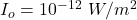We need to calculate the sound intensity in the distance of 20 m

Using formula of sound intensity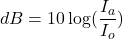Put the value into the formula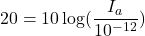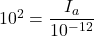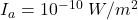If the conversation a sound level of 70.0 dB instead

We need to calculate the sound intensity

Using formula of sound intensity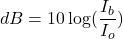Put the value into the formula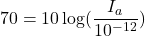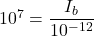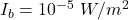We know that,

The intensity is inversely proportional with the square of the distance.

We need to calculate the distance

Using formula of intensity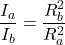Put the value into the formula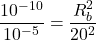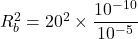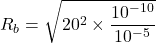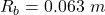Hence, The distance from the conversation should be 0.063 m.